How do amend a basic sum function not add a sub-table field based on another field?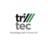I currently have a basic sum formula that totals all of the 'Item Total Net' fields within a sub-table as shown on the attached screen shots, however i would like a formula that omits any of the figures that have been ticked as a provisonal cost in a seperate field.

i have tried the follwing code but it keeps on indicating that there is a symbol missing after 'where', any suggetions?

sum(select 'Section 1 Items'.'Item Total (net)' where 'Section 1 Items'.'Provisional Cost' = 0)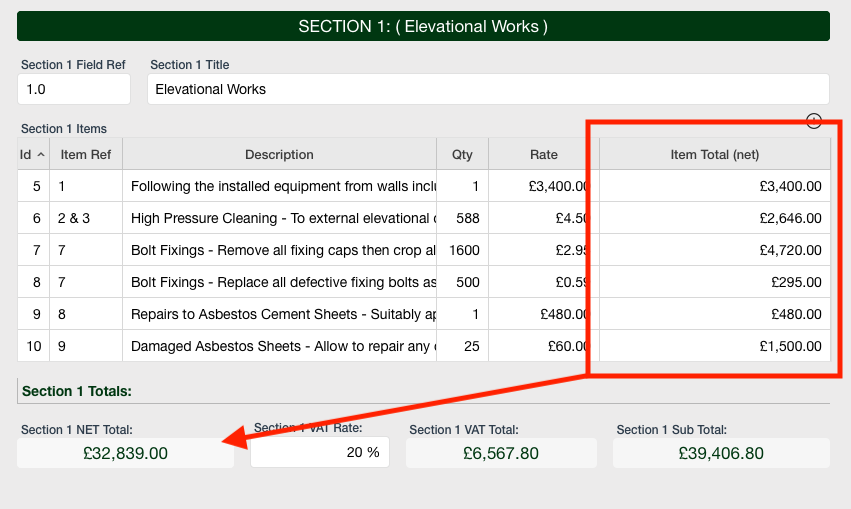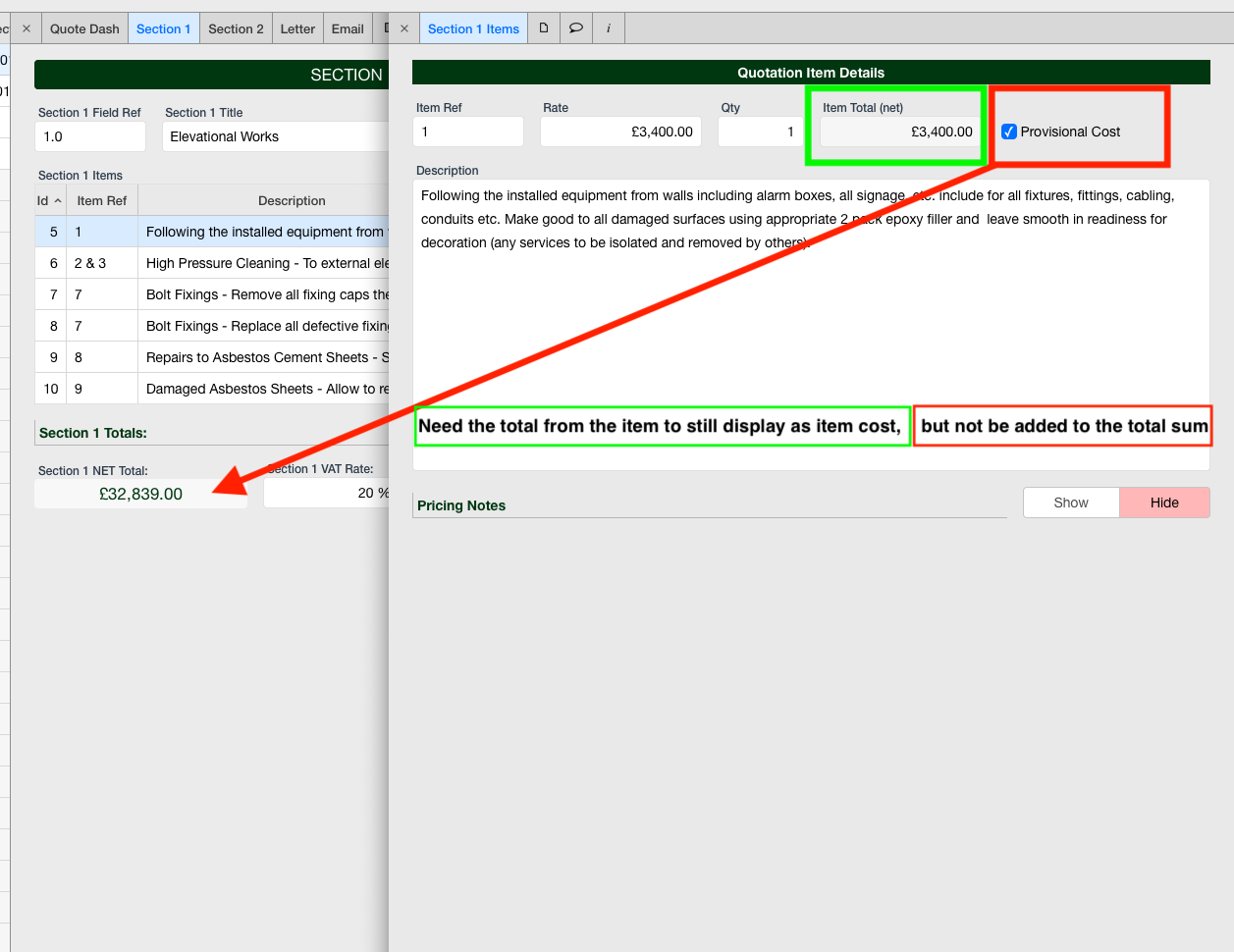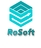Try:

sum(select 'Section 1 Items'.'Item Total (net)' where 'Provisional Cost' = "False")

Or (without the ")

sum(select 'Section 1 Items'.'Item Total (net)' where 'Provisional Cost' = False)

Steven.Great, i'll try that.Yeah it keeps on saying symbol expected at... which was what it was doing earlier? any ideas?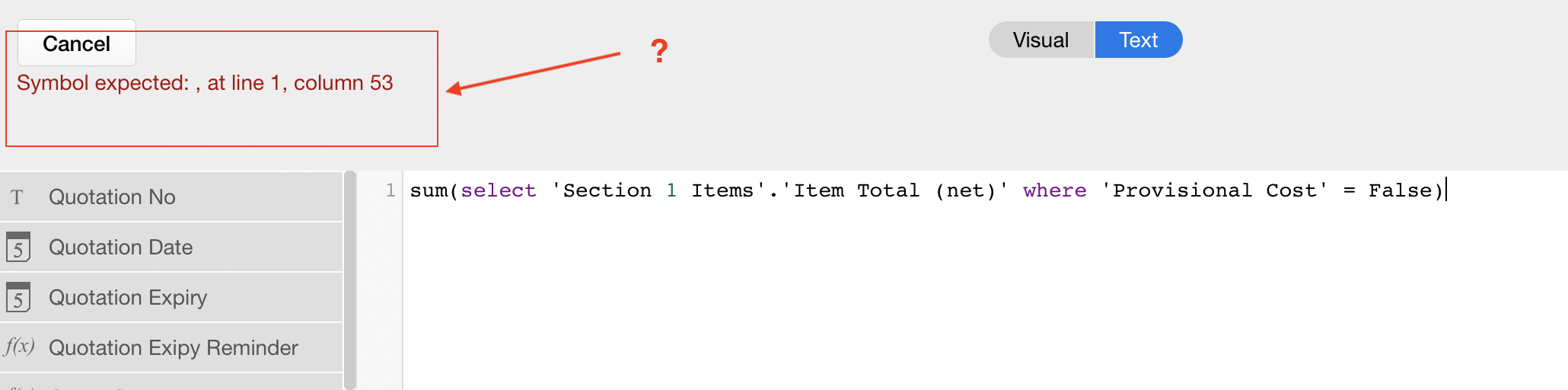which is directly after 'where'?Maybe this is Better:

---

let i := (select 'Section 1 Items' where 'Provisional Cost' = False);

sum(i.'Item Total (net)')

---

ps: can you try if this works also:

---

sum((select 'Section 1 Items' where 'Provisional Cost' = False).'Item Total (net)')

---

StevenNearly, but it adds the same amounts up for all the different quotes making all the sums for section one the accross all of them?*Makes the amounts in section one the same accross all the quote numbers.This might do it:

---

let me := this

let i := (select 'Section 1 Items' where 'Provisional Cost' = False and Id = me);

sum(i.'Item Total (net)')

---

StevenProblem solved, i ended up not too sure by luck (more of a process of elimination) adapting the follwing:

sum(select 'Section 1 Items'['Provisional Cost' = 0].'Item Cost Total') this as per previous formula's ending up selecting all the records within the table, literally adding up all the section 1 costs across all invoices, i just removed the 'select' statement and now it seems to be working seemlessly.

sum('Section 1 Items'['Provisional Cost' = 0].'Item Cost Total')

Thanks for all your help Steven.

1 2